• 像进行匹配，设计出 Haar 小波图像压缩和形状模板匹配相结合的算法及程序。最后，经大量实验结 果证实: 所提出的算法不仅匹配速度快，精度高，而且匹配结果不受遮蔽、混乱和非线性光照变化等 情况的影响，适宜于在...
• haar小波图像的一次分解实验，同样要求不使用matlab自带的小波函数和卷积函数。 分解过程： matlab代码如下： clc clear x=imread('cameraman.tif'); %读取matlab里自带的一副图片 %imshow(img);该图像的行列为...
haar小波对图像的一次分解实验，同样要求不使用matlab自带的小波函数和卷积函数。 分解过程：matlab代码如下：
clc
clear
%imshow(img);该图像的行列为366*364
%haar小波对图像进行分解

h1=x;
h3=x;
for i=1:256
for j=1:255
%对行,进行低通滤波 (1/2,1/2)
h1(i,1)=0+1/2*x(i,1);
h1(i,j)=1/2*x(i,j)+1/2*x(i,j+1);
%对行,进行高通滤波()
h3(i,1)=-1/2*x(i,1);
h3(i,j)=1/2*x(i,j)-1/2*x(i,j+1);
end
h1(i,256)=1/2*x(i,256);
end

for i=1:256
for j=1:128
%对行压缩
k=2*j;
h1(i,j)=h1(i,k);
h3(i,j)=h3(i,k);
end
end

h2=h1;
h4=h3;
for j=1:128
for i=1:255
%对列进行低通滤波
h1(1,j)=1/2*h1(1,j);
h1(i,j)=1/2*h1(i,j)+1/2*h1(i+1,j);
%对h2的列进行高通滤波
h2(1,j)=-1/2*h2(1,j);
h2(i,j)=1/2*h2(i,j)-1/2*h2(i+1,j);
%对h3的列低通滤波
h3(1,j)=1/2*h3(1,j);
h3(i,j)=1/2*h3(i,j)+1/2*h3(i+1,j);
%对h4的列高通滤波
h4(1,j)=-1/2*h4(1,j);
h4(i,j)=1/2*h4(i,j)-1/2*h4(i+1,j);
end
end

%对列压缩
for j=1:128
for i=1:128
k=2*i;
h1(i,j)=h1(k,j);
h2(i,j)=h2(k,j);
h3(i,j)=h3(k,j);
h4(i,j)=h4(k,j);
end
end

h1=h1(1:128,1:128);
h2=h2(1:128,1:128);
h3=h3(1:128,1:128);
h4=h4(1:128,1:128);

%haar小波的一次分解的边界太不明显，处理一下像素，使边缘更明显一些
n=9;
for i=1:128
for j=1:128
if(h2(i,j)*n<256)
h2(i,j)=n*h2(i,j);
end
if(h3(i,j)*n<256)
h3(i,j)=n*h3(i,j);
end
if(h3(i,j)*n<256)
h3(i,j)=n*h3(i,j);
end
if(h4(i,j)*n<256)
h4(i,j)=n*h4(i,j);
end
end
end

subplot(2,2,1),imshow(h1);
subplot(2,2,2),imshow(h2);
subplot(2,2,3),imshow(h3);
subplot(2,2,4),imshow(h4);


计算结果如下图所示，由于Haar小波的一次分解图像边缘太不明显，于是手动把像素计算结果放大了。展开全文matlab
• 鉴于小波多尺度分解与重构在图像的编码、压缩、去燥、融合等方面的重要作用，介绍了Haar 小波的尺度函数与小波函数，给出了Haar 小波多尺度分解与重构的算法，并进行成功验证。结果表明，多尺度分解将图像分解成一个...
• 基于Haar小波的小波离散变换作为数据压缩小波技术和基于收缩线性变换系统的分形技术之间的纽带。matlab
• ## haar小波的举例

千次阅读 2018-07-31 15:27:11
小波变换的基本思想是用一组小波函数或者基函数表示一个函数或者信号，例如图像信号。为了理解什么是小波变换，下面用一个具体的例子来说明小波变换的过程。假设有一幅分辨率只有4个像素的一维图像，对应的像素值...
小波变换的基本思想是用一组小波函数或者基函数表示一个函数或者信号，例如图像信号。为了理解什么是小波变换，下面用一个具体的例子来说明小波变换的过程。假设有一幅分辨率只有4个像素的一维图像，对应的像素值分别为[9 7 3 5]   计算它的哈尔小波变换系数:  (1).求均值(averaging)。计算相邻像素对的平均值，得到一幅分辨率比较低的新图像，它的像素数目变成了2个，即新的图像的分辨率是原来的1/2，相应的像素值为：[8 4] (2).求差值(diqqerencing)。很明显，用2个像素表示这幅图像时，图像的信息已经部分丢失。为了能够从由2个像素组成的图像重构出由4个像素组成的原始图像，就需要存储一些图像的细节系数,以便在重构时找回丢失的信息。方法是把像素对的第一个像素值减去这个像素对的平均值，或者使用这个像素对的差值除以2。在这个例子中，第一个细节系数是(9-8)=1，因为计算得到的平均值是8,它比9小1而比7大1，存储这个细节系数就可以恢复原始图像的前两个像素值。使用同样的方法，第二个细节系数是(3-4)=-1，存储这个细节系数就可以恢复后2个像素值。因此，原始图像就可以用下面的两个平均值和两个细节系数表示[8 4 1 -1] (3).重复第1，2步，把由第一步分解得到的图像进一步分解成分辨率更低的图像[6 2 1 -1].   由此可见，通过上述分解就把由4像素组成的一幅图像用一个平均像素值和三个细节系数表示，这个过程就叫做哈尔小波变换,也称哈尔小波分解(Haar wavelet decomposition)。这个概念可以推广到使用其他小波基的变换。在这个例子中我们可以看到，①变换过程中没有丢失信息，因为能够从所记录的数据中重构出原始图像。②对这个给定的变换，我们可以从所记录的数据中重构出各种分辨率的图像。例如，在分辨率为1的图像基础上重构出分辨率为2的图像，在分辨率为2的图像基础上重构出分辨率为4的图像。③通过变换之后产生的细节系数的幅度值比较小，这就为图像压缩提供了一种途径，例如去掉一些微不足道的细节系数而不影响对重构图像的理解。   求均值和差值的过程实际上就是一维小波变换的过程，现在用数学方法重新描述小波变换的过程。
个人理解：原本以为小波变换后得到的低频系数与高频系数分别会与原始数据长度一致，其实在一级分解的情况下，一半是低频系数一半是高频系数；而二级的是将一级变换后的低频数据进行再次变换；
【9，7，3，5】为原始数据，一级低频数据为 【8，4】，二级低频以一级低频数据为原始数据，得到【6】；而一级高频数据为【1，-1】，二级高频数据为【2，1，-1】；整个变换流程：【9，7，3，5】转换为低频【8，4】+高频【3，5】；而二级变换为将一级中的低频进行变换，【8，4】转换成【6】与高频的【2】；所以最终结果为：最终低频【6】+一级高频【1，-1】+二级高频【2】，即【6，2，1，-1】
展开全文• 图像进行Haar小波变化。 运行时参数： 文件名 选择的像素点个数
• 小波场的相关匹配技术中，由于小波的垂直分量和水平分量反映了图像的边缘等高频信息，所以分解后的小波特征场相关性小，有比较高的匹配精度；同时，由于数据量压缩，提高了匹配速度，适应于地形匹配的快速实现。
• 实现了彩色图像Haar小波的分解与重构与灰度图像的haar小波分解与重构，图像压缩、图像去噪、图像增强等功能，界面美观，交互美好，使用MATLAB软件
• 我试图在python中的图像上应用haar小波。这是代码from pywt import dwt2, idwt2img = cv2.imread('xyz.png')cA, (cH, cV, cD) = dwt2(img, 'haar')然后我修改嵌入一些数据的系数，如下所示cH1=cH+k*pn_sequence_hcV1...
我试图在python中的图像上应用haar小波。这是代码from pywt import dwt2, idwt2
cA, (cH, cV, cD) = dwt2(img, 'haar')
然后我修改嵌入一些数据的系数，如下所示cH1=cH+k*pn_sequence_h
cV1=cV+k*pn_sequence_v
之后，我将idwt应用于下面的代码idwt2(cA,(cH1,cV1,cD),'haar')[:Mc,:Nc]
其中Mc和Nc是分解图像的高度和宽度。
但是，我收到此代码的错误。以下是错误。Traceback (most recent call last):
File "dwt.py", line 15, in
idwt2(cA,(cH1,cV1,cD),'haar')[:Mc,:Nc]
File "C:\Python27\lib\site-packages\pywt\_multidim.py", line 104, in idwt2
LL, (HL, LH, HH) = coeffs
ValueError: too many values to unpack
我该怎么做才能解决这个错误？我是python的新手。任何帮助将不胜感激。
我也在下面的代码中尝试过这样的。但在这里我没有得到像CA，CH，CV，CD这样的系数。我得到的是所有系数。import numpy as np
import pywt
import numpy
import PIL
from PIL import Image
img = PIL.Image.open("rot.png").convert("L")
imgarr = numpy.array(img)
coeffs = pywt.dwt2(imgarr, 'haar')
pywt.idwt2(coeffs, 'haar')
展开全文• 小波变换的基本思想是用一组小波函数或者基函数表示一个函数或者信号，例如图像信号。首先，以haar小波变换过程为例来理解小波变换。 例：求有限信号的均值和差值 假设有一幅分辨率只有4个像素 的一维图像，对应的...
小波可以认为是一个带通滤波器，只允许频率和小波基函数频率相近的信号通过。小波变换的基本思想是用一组小波函数和基函数表示一个函数或者信号。
haar小波变换
首先，以haar小波变换过程为例来理解小波变换。
例：求只有4个像素[9 7 3 5]的图像的哈尔小波变换系数。 计算步骤如下：
步骤1：求均值(averaging)，也叫Approximation。计算相邻像素对的平均值，得到一幅分辨率比较低的新图像，新的图像的分辨率是原来的1/2，相应的像素值为：[8 4]
步骤2：求差值(differencing)。很明显，用2个像素表示这幅图像时，图像的信息已经部分丢失。为了能够从由2个像素组成的图像重构出由4个像素组成的原始图像，就需要存储一些图像的细节系数(detail coefficient)，以便在重构时找回丢失的信息。方法是使用这个像素对的差值除以2，结果为[8 4 1 -1]
步骤3：重复第1，2步，把由第一步分解得到的图像进一步分解成分辨率更低的图像和细节系数。[6 2 1 -1]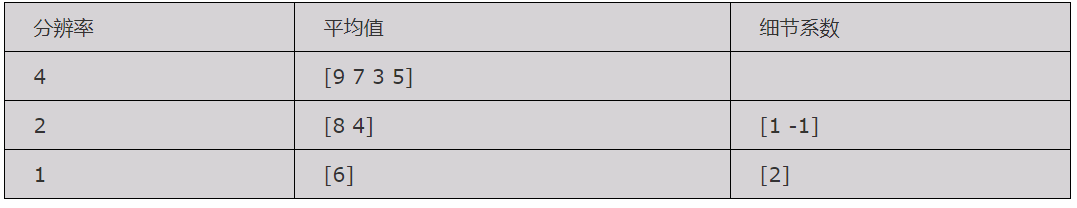从这个例子中我们可以看到： ① 对这个给定的变换，我们可以从所记录的数据中重构出各种分辨率的图像。例如，在分辨率为1的图像基础上重构出分辨率为2的图像，在分辨率为2的图像基础上重构出分辨率为4的图像。 ②变换过程中没有丢失信息，因为能够从所记录的数据中重构出原始图像。 ③ 通过变换之后产生的细节系数的幅度值比较小，这就为图像压缩提供了一种途径，例如去掉一些微不足道的细节系数并不影响对重构图像的理解。
一维离散小波变换
经过小波变换后图像会生成低频信息和高频信息。低频信息对应于求均值，高频信息对应于求差值。 均值是局部的平均值，变化缓慢，属于低频信息，存储图片的轮廓信息，近似信息 差值是局部的波动值，变化较快，属于高频信息，存储图片的细节信息，局部信息，另外含有噪音

h

h

i

g

h

h_{high}

是高通滤波器，允许高频信息通过

h

h

i

g

h

h_{high}

是低通滤波器，允许低频信息通过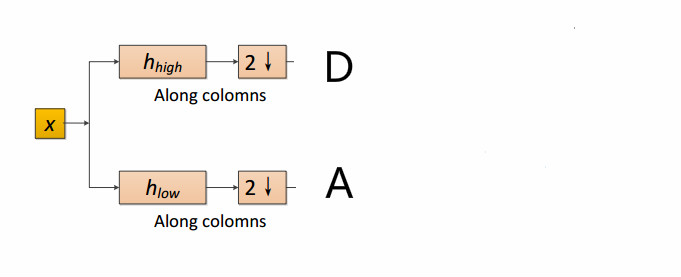在haar小波中，

h

h

i

g

h

=

(

1

2

,

−

1

2

)

h_{high}=(\frac{1}{\sqrt{2}},-\frac{1}{\sqrt{2}})

，

h

l

o

w

=

(

1

2

,

1

2

)

h_{low}=(\frac{1}{\sqrt{2}},\frac{1}{\sqrt{2}})

import pywt
data = [1, 1, 1, 1]
coeffs = pywt.dwt(data, 'haar')
# (cA, cD) : tuple   Approximation and detail coefficients.
cA, cD = coeffs

cA:[1.41421356 1.41421356]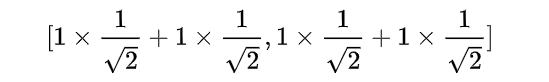cD:[0. 0.]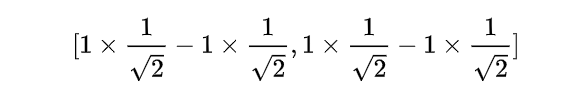二维离散小波变换

h

h

i

g

h

h_{high}

是高通滤波器，允许高频信息通过

h

h

i

g

h

h_{high}

是低通滤波器，允许低频信息通过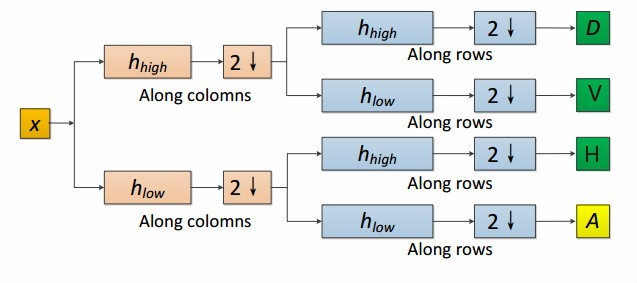A是低频信息，H是水平高频信息，V是垂直高频信息、D是对角高频信息。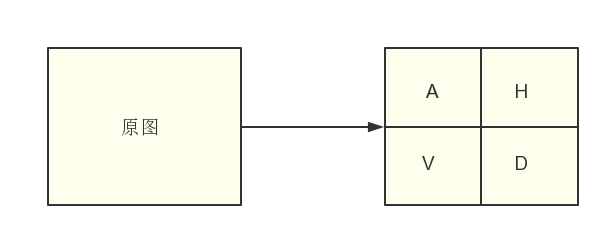import numpy as np
import pywt
data = np.ones((4, 4), dtype=np.float64)
[[1. 1. 1. 1.]
[1. 1. 1. 1.]
[1. 1. 1. 1.]
[1. 1. 1. 1.]]

coeffs = pywt.dwt2(data, 'haar')
# (cA, (cH, cV, cD)) : tuple
# Approximation, horizontal detail, vertical detail and diagonal
# detail coefficients respectively
cA, (cH, cV, cD) = coeffs

print(cA)
[[2. 2.]
[2. 2.]]

print(cH)
[[0. 0.]
[0. 0.]]


假设一张图片只有4个像素，其经过2-D DWT之后得到4张子图，每个子图的详细计算过程如下：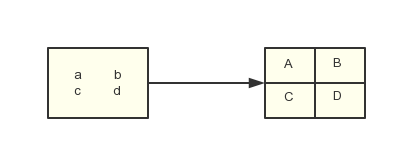A

=

1

2

(

a

+

b

+

c

+

d

)

A=\frac{1}{2}(a+b+c+d)

B

=

1

2

(

a

−

b

+

c

−

d

)

B=\frac{1}{2}(a-b+c-d)

C

=

1

2

(

a

+

b

−

c

−

d

)

C=\frac{1}{2}(a+b-c-d)

D

=

1

2

(

a

−

b

−

c

+

d

)

D=\frac{1}{2}(a-b-c+d)

更多相关资料： 小波变换入门参考资料 [Python ]小波变化库——Pywalvets 
展开全文• 去年11月发布了一系列有关小波变换和图像处理的文章，把学习小波过程中的心得体会和编写的程序放在网上和大家共享交流...有关小波图像分解和重构的两篇文章中分享的程序，存在下列问题： （1）程序所用的小波函数只有
• 通过实验得出了小波变换及haar小波用于图像分解与重构具有速度快、重构图像压缩比高、对图像容易进行各种分析处理等优点的结论,为后续研究图像压缩技术提供了一定的依据。 关键词:小波变换 分解 重构 压缩
• 介绍了小波变换的分解与重建，提出了包括IVIW光照补偿方法、基于Haar小波的去噪与压缩方法，并通过MATLAB R2009a平台对Yale人脸库中的图像进行成功验证。结果表明，基于小波变换的图像预处理方法简单易行且有效，...
• matlab开发-小波压缩。基于HAAR小波变换的RGB和灰度图像压缩
• 此次任务参考了以下大佬的博客，链接： haar小波： https://blog.csdn.net/baidu_27643275/article/details/84826773 【小波变换】小波变换python实现–PyWavelets： ...python代码： import numpy
• ## 【转】小波图像分解与重构

万次阅读 多人点赞 2018-02-10 16:31:14
原文地址：【转】小波图像分解与重构作者：freedesert小波图像融合综述（1）分类： 小波变换2007-11-14 11:15 4728人阅读 评论(6) 收藏 举报 图像融合是将两幅或多幅图像融合在一起，以获取对同一场景的更为...
• 目 录摘 要 1Abstract 11、 绪论 21.1 课题开发背景 21.1.1 图像融合的定义 21.1.2 手动配准与图象融合 21.1.3 图象融合研究的发展现状和研究热点 31.2 课题设计要求 42、MATLAB程序设计 52.1 MATLAB软件简介 52.2 ...算法 大数据 编程语言 python 机器学习
• 该程序使用小波进行压缩和重构。 我们可以使用选定的小波选择图像的分解级别（这里最多给出 4 个级别）。 例如：-wavelets 可以是 haar、db1、db2、dmey………… 分解可以看图。 （请注意选择 256X256 图像以获得更...matlab
• 小波变换实现图像压缩 代码 X=imread('a5.jpg'); X=rgb2gray(X); subplot(221); imshow(X); title('原始图像'); %对图像用小波进行层小波分解 [c,s]=wavedec2(X,2,'haar'); %提取小波分解结构中的一层的低频系数和...MATLAB 数字图像处理
• 小波变换在图像压缩方面的实现与应用一、实验图片的基本信息二、数据处理过程2.1小波函数的选择2.2图像压缩的基本思想三、不同小波函数压缩程度的对比四、MATLAB源码 一、实验图片的基本信息 小波变换作为一种新的...matlab
• 1974年，法国工程师J.Morlet首先提出小波变换的概念，1986年著名数学家Y.Meyer偶然构造出一个真正的小波基，并与S.Mallat合作建立了构造小波基的多尺度分析之后，小波分析才开始蓬勃发展起来。小波分析的应用领域...计算机视觉 人工智能 python 算法 matlab
• 最近，小波变换已成为图像压缩和水印应用的流行技术。 小波变换具有很高的去相关性和能量压缩效率。 本文介绍了各种小波水印技术的比较研究，并在水印图像质量、提取水印质量及其对 JPEG 2000 压缩的实质方面测试了...
• 我们在本文中讨论了不同的小波，例如 Symlet、Haar、Biorthogonal、Daubechies，并使用张量分解来比较多媒体应用视频压缩的性能分析。 四种不同的基于小波压缩方法，如 SPIHT-3D、STW & EZW、SPIHT-3D 和 SPIHT，...
• 图像融合（六）-- 小波融合。 这是一篇关于小波融合的图像融合算法，供自己以后的学习。感谢此篇文章作者的付出。 http://blog.sina.com.cn/s/blog_149e9d2ec0102wxv9.html
• 小波降噪的方法有多种，如利用小波分解与重构的方法滤波降噪、利用...其中最常用的就是阈值法去噪，其基本思想就是利用图像小波分解后，各个子带图像的不同特性选取不同的阈值，从而达到较好的降噪目的。一、小波降...
• 另外参见俄罗斯写的... 一、Haar小波的基本原理  数学理论方面的东西我也不是很熟悉，这边主要用简单的例子来介绍下Haar小波的使用情况。...